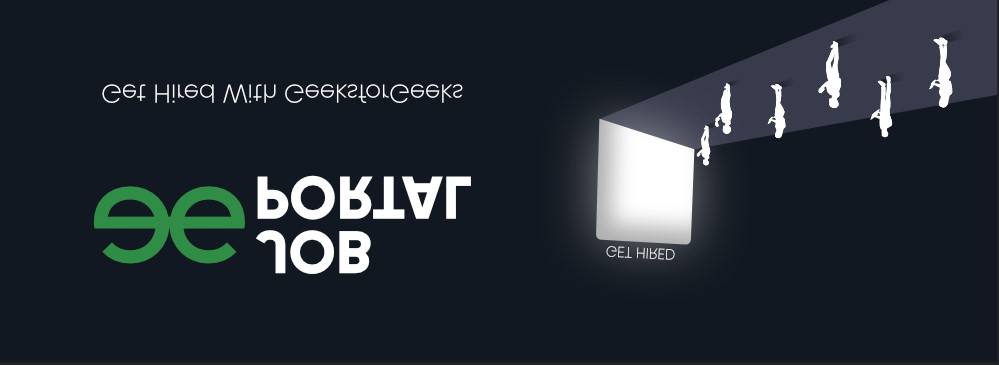Open in App
Not now

# RandomVerticalFlip() Method in Python PyTorch

• Last Updated : 03 Jun, 2022

In this article, we are going to discuss RandomVerticalFlip() Method in PyTorch using Python.

## RandomVerticalFlip() Method

RandomVerticalFlip() method of torchvision.transforms module is used to vertically flip the image at a random angle with a given probability.  This accepts a PIL image and a tensor image as an input. The tensor image is a PyTorch tensor with [C, H, W] shape, where C represents the number of channels and  H, W represents the height and width respectively. This method returns a randomly flipped image.

Syntax: torchvision.transforms.RandomVerticalFlip(p)

Parameter:

• p: p is the probability of the image being flipped.
• img: input image.

Returns: This method returns a randomly flipped image. This method returns vertically flipped image if P=1, returns original image if P=0 and if p in between the range of 0 to 1 then P is the probability to return the vertically flipped image.

The below image is used for demonstration:Example 1:

The following program is to understand the RandomVerticalFlip() Method when the probability is 1.

## Python3

 `# import required libraries ` `import` `torch ` `import` `torchvision.transforms as T ` `from` `PIL ``import` `Image ` ` `  `# read input image from computer ` `img ``=` `Image.``open``(``'a.png'``) ` ` `  `# define a transform ` `transform ``=` `T.RandomVerticalFlip(p``=``1``) ` ` `  `# apply above defined transform to ` `# input image ` `img ``=` `transform(img) ` ` `  `# display result ` `img.show() `

Output:Example 2:

The following program is to understand the RandomVerticalFlip() Method when the probability is in the range of 0 to 1.

## Python3

 `# import required libraries ` `import` `torch ` `import` `torchvision.transforms as T ` `from` `PIL ``import` `Image ` ` `  `# read input image from computer ` `img ``=` `Image.``open``(``'a.png'``) ` ` `  `# define a transform ` `transform ``=` `T.RandomVerticalFlip(p``=``0.5``) ` ` `  `# apply above defined transform to  ` `# input image ` `img ``=` `transform(img) ` ` `  `# display result ` `img.show() `

Output:My Personal Notes arrow_drop_up
Related Articles